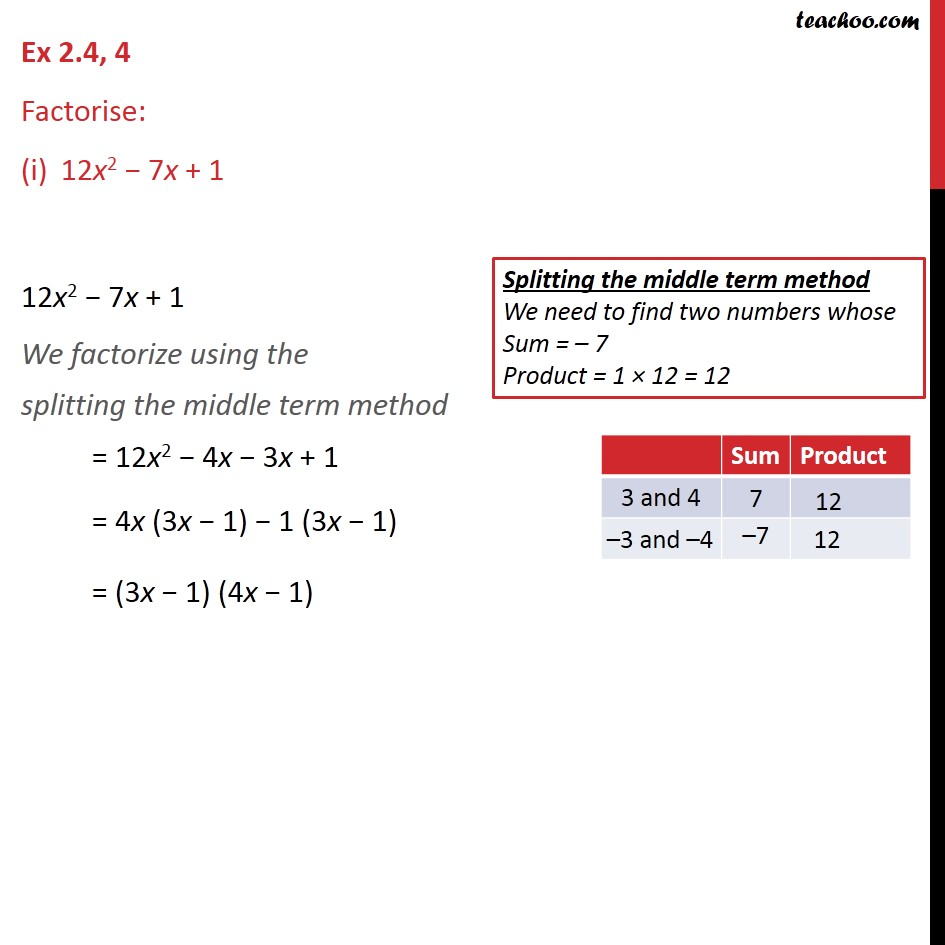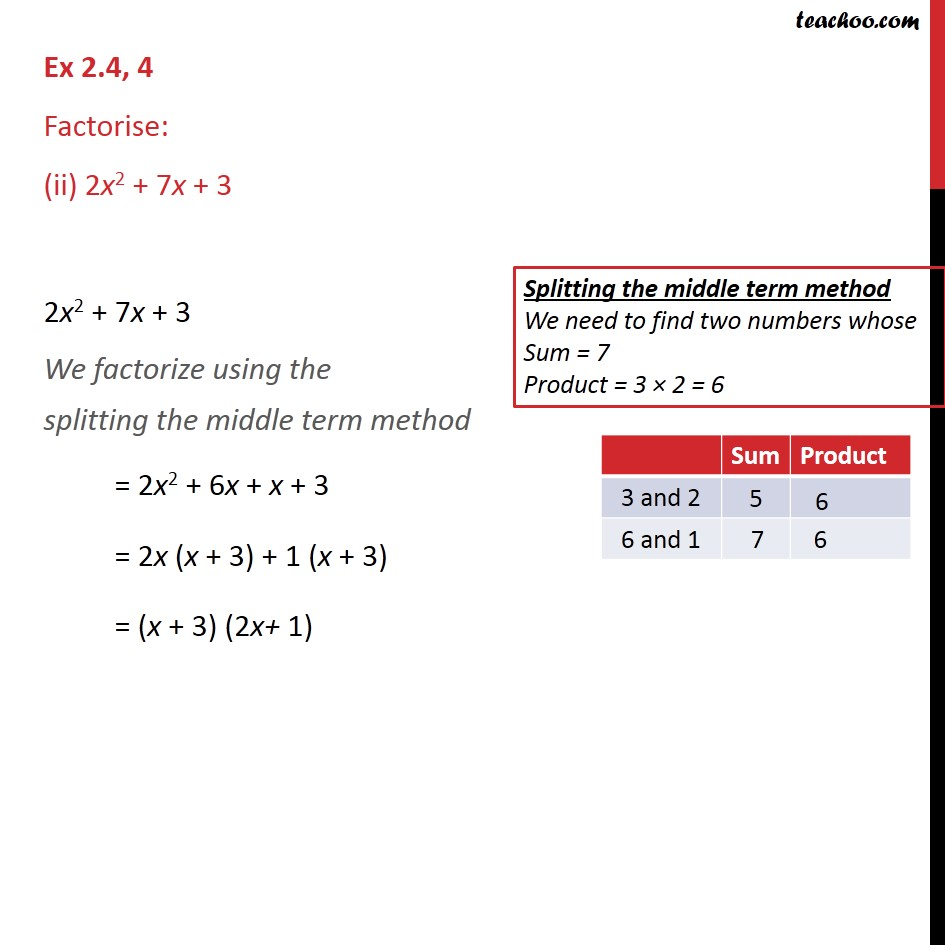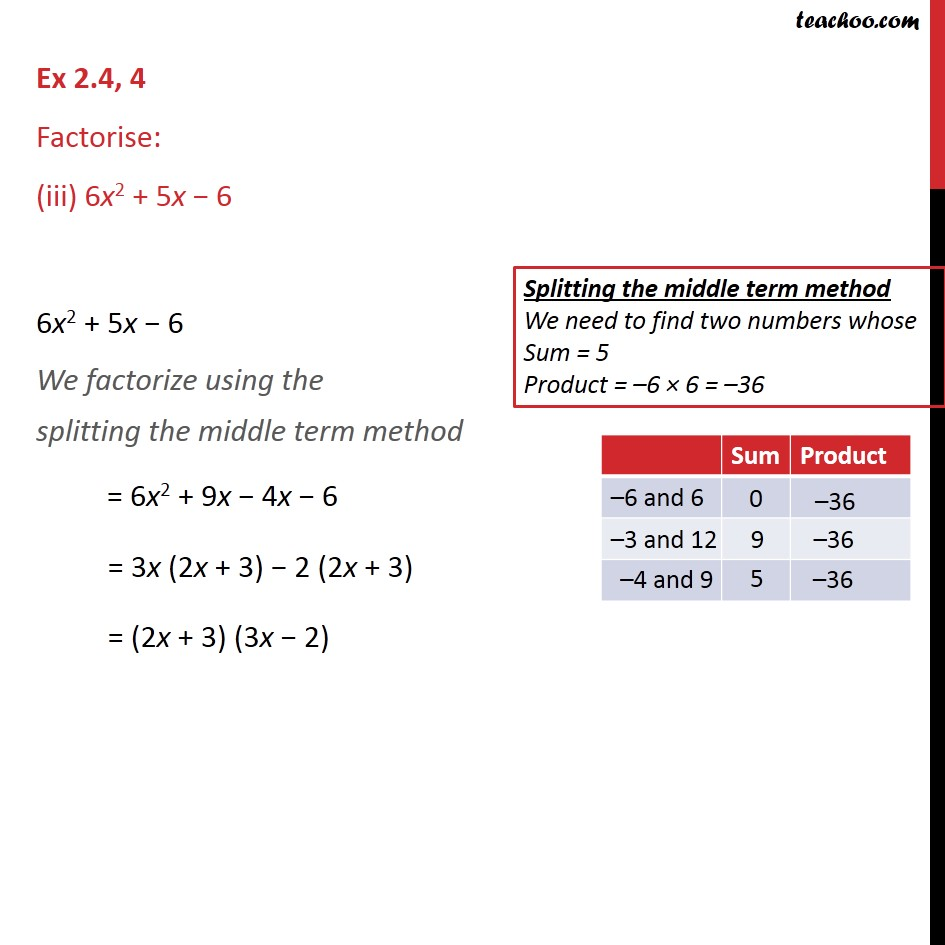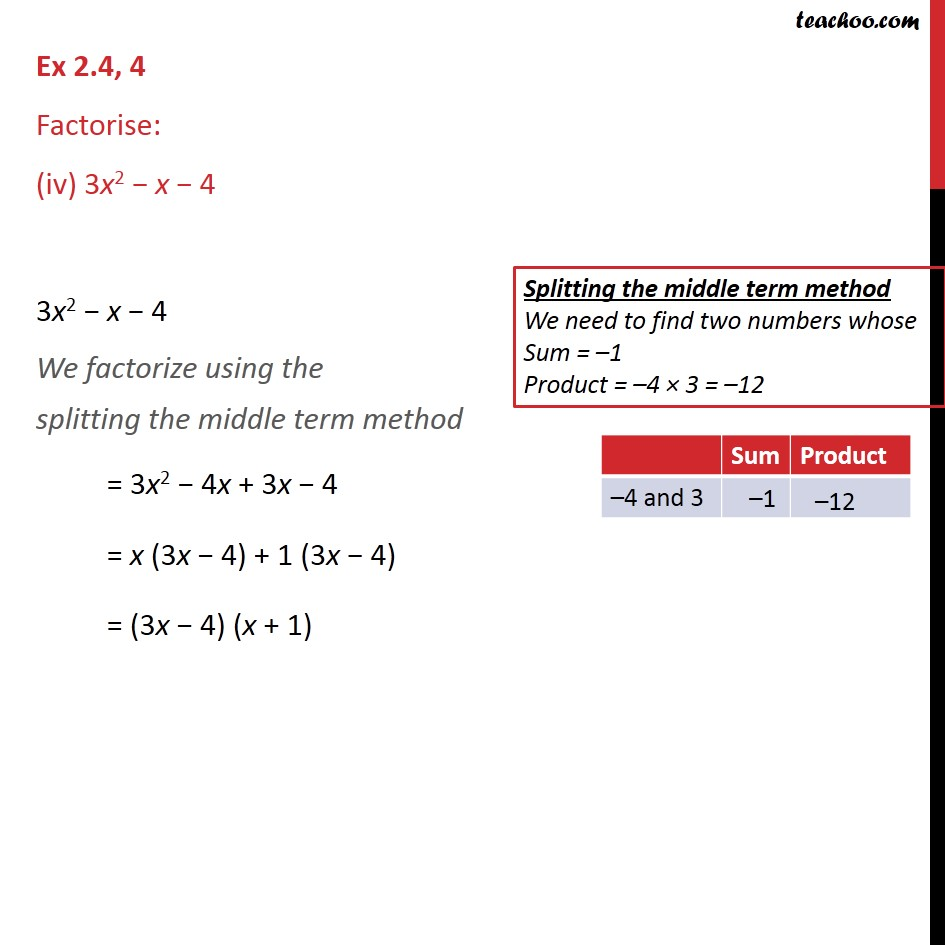1. Chapter 2 Class 9 Polynomials (Term 2)
2. Serial order wise
3. Ex 2.4

Transcript

Ex 2.4, 4 Factorise: 12x2 7x + 1 12x2 7x + 1 We factorize using the splitting the middle term method = 12x2 4x 3x + 1 = 4x (3x 1) 1 (3x 1) = (3x 1) (4x 1) Ex 2.4, 4 Factorise: (ii) 2x2 + 7x + 3 2x2 + 7x + 3 We factorize using the splitting the middle term method = 2x2 + 6x + x + 3 = 2x (x + 3) + 1 (x + 3) = (x + 3) (2x+ 1) Ex 2.4, 4 Factorise: (iii) 6x2 + 5x 6 6x2 + 5x 6 We factorize using the splitting the middle term method = 6x2 + 9x 4x 6 = 3x (2x + 3) 2 (2x + 3) = (2x + 3) (3x 2) Ex 2.4, 4 Factorise: (iv) 3x2 x 4 3x2 x 4 We factorize using the splitting the middle term method = 3x2 4x + 3x 4 = x (3x 4) + 1 (3x 4) = (3x 4) (x + 1)

Ex 2.4